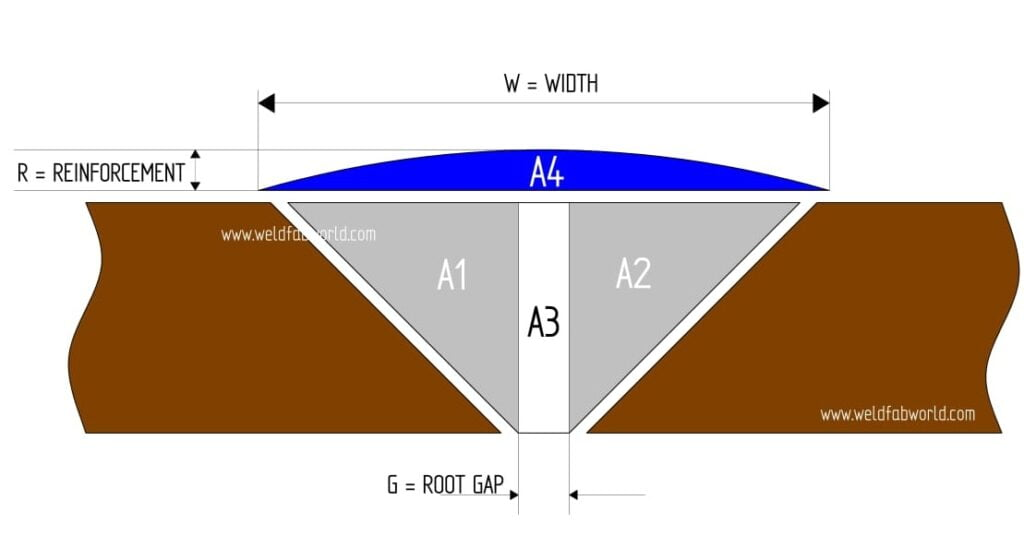# WeldFabWorld Calculator

## Pipe Weight Calculator

Weight = Volume x Material DensityOD = Outside Diameter of PipeID = Inside Diameter of PipeID = OD – 2TT = Thickness of Pipe L=Length of Pipe Volume of Pipe = π⁄4 (OD²-ID²) x L Weight of Pipe = π⁄4 (OD²-ID² ) x L x Material Density

## Hemispherical Dishend Weight Calculator## Elbow Weight Calculation Calculator

Elbow is the most commonly used fitting  to change the angle or direction of the pipe run. Most commonly in 90 degrees and 45 degree turns. Knowing the approximate weight of the fittings helps us calculate the: Welding consumable cost for cladding Price calculation of item Freight charge of the items O.D= Outside Diameter of …## Weld Consumable Calculation for “Single V Groove joint”

This article is regarding the benefits of weld consumable calculation. Steps to calculate weld consumable & we will calculate weld consumable for single “V” groove joint.  In welding, weld consumable estimation plays the vital role for complete the fabrication within time frame and at the possible minimum cost of weld metal as other cost of …